### TOPIC 7 – FORMULA BONDING AND NOMENCLATURE

ATOMIC STRUCTURE
TOPIC 5: ATOMIC STRUCTURE
We learned early that matter is made up of small, indivisible particles. Everything around us is made of extremely small particles. These particles are either molecules or atoms. An atom is the smallest indivisible particle of an element that takes part in a chemical change. Atoms are the building blocks of matter. All solids, liquids and gases are made of atoms fitted in different ways.

The present day chemistry is built on the foundations of the Atomic Theory. The idea that elements are made up of atoms is called the Atomic Theory. An English chemist, John Dalton was the first to put forward the Atomic Theory, which for most of the 19th century stated that atoms were hard, extremely small, indivisible and spherical particles like minute lead shots

Dalton Contribution to Atomic Structure
Explain Dalton contribution to atomic structure

The Greek philosopher Democritus (460-370 BC) believed that matter was indestructible and that it is made up of tiny particles called atoms. Our modern understanding is based on the Atomic Theory which was put forward by John Dalton in 1808. His theory re-introduced the ideas of Democritus and other Greek philosophers who suggested that all matter was infinitely divided into very small particles called atoms. These ideas were not widely accepted at that time. They were only revived when Dalton developed them further and experimental science was able to back them up with practical observations.

The Atomic Theory goes back to ancient Greeks, yet we always talk today about Dalton’s Atomic Theory. There is a good reason for this. The reason is that, while Greeks put forward the idea that atoms exist they did nothing more. They left the idea vague and untested. Dalton changed this vague imaging into a set of concrete suggestions about atoms which could be tested by experiment. This change from vagueness to precision and experimental test justifies his claim to the theory.

Dalton’s Atomic Theory contains the following main ideas:
1. Matter is made up of small, indivisible particles called atoms.
2. Atoms of the same element are all exactly alike in every way and have definite weights.
3. Atoms are indestructible and they cannot be created.
4. Atoms of different elements have different weights and posses different properties.
5. Atoms of different elements combine in small whole numbers to form ‘compound atoms’.

The Modern Concept of Dalton’s Atomic Structure
Explain the modern concept of Dalton’s atomic structure

From the theory, it is observed that each atom has its own mass and that chemical combination takes place between atoms and not fractions of atoms.

Discoveries made in the 20th century, however, showed that certain parts of the theory must be modified. However, Dalton‟s Theory was one of the great leaps of understanding of chemistry. It meant that we could explain many natural processes.

Dalton’s Atomic Theory was the first step towards the formation of Modern Atomic Theory. The Dalton’s Theory has been subjected to numerous experimentations that have led to some modifications to the theory. However, some ideas in his theory still hold strongly to date. Some modifications to the theory include the following:
1. The atom is no longer regarded as indivisible, or the smallest particle. Particles smaller than the atom; electrons, protons and neutrons are now known. However, the atom is still the smallest particle which can take part in a chemical reaction.
2. Atoms of the same element may not be all alike. Some elements have atoms with different atomic masses e.g. carbon 12 and carbon 14. These different atoms of the same element are called isotopes.
3. In some few cases, atoms of different elements may have the same atomic mass. Both argon and calcium have atomic mass 40. Such atoms are called isobars.
4. “The compound atoms” of Dalton are known as molecules. A molecule is the simplest particle of matter which is capable of independent existence. Evidence is available where atoms of different elements combine in large integers. An example is in organic and silicon compounds.
5. Atoms are no longer regarded as indestructible. Radioactive atoms may get destroyed by spontaneous decay or by atomic fission.The atom is therefore the smallest particle of an element which is responsible for the chemical properties of that element, and which takes part in a chemical reaction.

Sub-atomic Particles in an Atom
Identify sub-atomic particles in an atom

Dalton thought that atoms were solid, indivisible particles. But, as a result of work done mainly by Lord Rutherford, the idea has been greatly changed in recent years. According to Rutherford, the atom consists of 3 kinds of particles – protons, neutrons and electrons. These are called sub-atomic particles.

The centre of the atom is called nucleus. The nucleus contains a cluster of two sorts of particles, protons and neutrons. The nucleus is very small, occupying only about 1% of the volume of an atom. The rest of the atom is mostly empty space, with electrons spread out in it.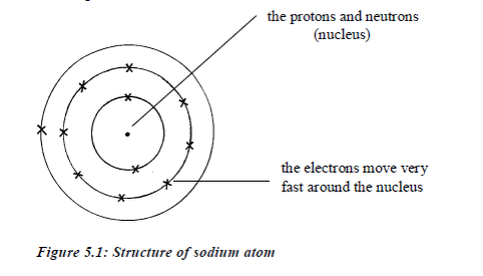Electrons move around the nucleus in special paths called electron shells (orbits/or orbitals or energy levels). Protons and electrons have electric charges. Neutrons have no charges.All the particles in an atom are very light. Their masses are measured in atomic mass units rather than grams. The proton isa positively charged particle. Its mass is about equal to that of hydrogen atom. The neutron is has no charge, it is neutral. Its mass is about equal to that of hydrogen atom. The electron is negatively charged. Its charge is equal but opposite to the charge on the proton. It has a very small mass, about 1⁄1840 of the mass of the proton.

The Properties of each Particle in an Atom
Explain the properties of each particle in an atom

The properties of these particles are summarized in the table below:

Table 5.1: Properties of sub-atomic particles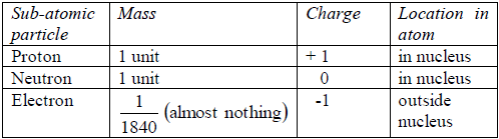A single atom is electrically neutral (it has no electrical charge).This means that in any atom there must be equal numbers of protons and electrons. In this way, the total positive charge on the protons is balanced by the total negative charge on the electrons orbiting the nucleus. So, the charges must cancel.
Electronic arrangement refers to the manner in which electrons are arranged in an atom. An atom contains a central nucleus containing protons and neutrons, and a cluster of electrons revolving in orbits around the nucleus. These electrons are grouped in shells.

A Maximum Number of Electrons in the Shells
Determine a maximum number of electrons in the shells

Bohr (1913) put forward a theory of electron positioning whichis still generally accepted and used until now for chemicalpurposes. Bohr’s Theory on the arrangement of electrons in anatom can be summarized as follows:
• Electrons are in orbit around the nucleus of the atom.
• The electron orbits are grouped together in shells; a shell is a group of orbits occupied by electrons with approximately equal energy.
• The electrons in shells distant from the nucleus have higher energy than those in shells close to the nucleus.
• Electrons fill the shells starting with the first shell, which is closest to the nucleus. Shells are numbered 1, 2, 3, 4,98etc. outwards from the nucleus. The shells may be represented by the letters K, L, M and N respectively starting from the nucleus.
• The maximum possible number of electrons in a shell numbered n is 2 2n .
• The first shell can only contain up to 2 electrons. The second shell can contain a maximum of 8 electrons. The third shell can contain up to 18 electrons.
• In the outermost shell of any atom, the maximum number of electrons possible is 8.
• The outer electrons of some atoms can be removed fairly easily to form ions.
• Chemical bonding between atoms to form molecules involves the electrons in the outer shell only.

Electronic arrangement of some typical atoms is shown in figure 5.2.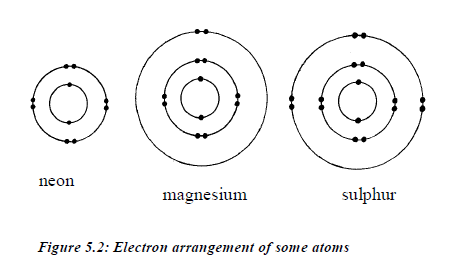The arrangement of electrons around the nucleus is also known as electronic configuration. This arrangement depends on the maximum number of electrons that can occupy a shell. An atom with 13 electrons will have the following electronic configuration: 2:8:3. This means that there are 2 electrons in the first shell, 8 electrons in the second shell and 3 electrons in the third shell.

The number and arrangement of electrons in the atoms of the first 20 elements are shown in table 5.2.

Table 5.2: The electron arrangements of the first 20 elements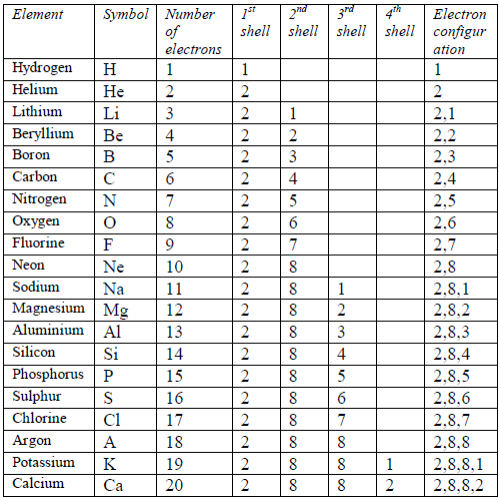After the first 20 elements, the organization of the electrons becomes increasingly complicated. The third shell (n = 3) can be occupied by a maximum number of 18 electrons

At this stage, you will not be asked to work out electron arrangements beyond element 20 (calcium), but you should be able to understand the electronic structures involving more electrons (for example bromine with the arrangement 2:8:18:7).

Energy Shell Diagrams
Draw energy shell diagrams
Energy Shell Diagram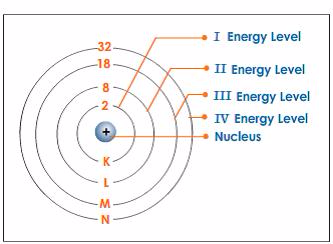Relationship between Atomic Number and Number of Protons
Relate atomic number with number of protons
All atoms of one element have the same number of protons. This is called the atomic number (or proton number) of that element.It is given by the symbol Z.

No two elements can have the same atomic number. Sodium atoms have 11 protons. This is what makes them different from all other atoms. Only sodium atoms have 11 protons, and any atom with 11 protons must be sodium atom.

In the same way, an atom with 6 protons must be carbon atom.Also any atom with 7 protons must be nitrogen atom. So, you identify an atom by the number of protons in it. There are 109elements altogether. Of these, hydrogen has the smallest atoms, with only 1 proton each. Helium atoms have 2 protons each.Lithium atoms have 3 protons each, and so on up to meitnerium atoms, which have 109 protons each. Table 5.3 shows the first20 elements arranged according to the number of protons they have.

Every atom has an equal number of protons and electrons, so the atomic number also tells us the number of electrons in that atom.In any given atom of an element, the number of neutrons has no effect on the identity and properties of that particular element. It is the number of protons and electrons that determine the identity and properties of any given element. The number of neutrons only affects the mass, since each one of them has the same mass as that of a proton.

Mass Number of an Atom from Numbers of Protons and Neutrons
Calculate mass number of an atom from numbers of protons and neutrons

Protons alone do not make up all the mass of an atom. The neutrons in the nucleus also contribute to the total mass. The mass of the electrons can be regarded as so small that it can be ignored. As a proton and a neutron have the same mass, the mass of a particular atom depends on the total number of protons and neutrons present. This is called mass number (or nucleon number). The mass number of an atom is found by adding together the number of protons and neutrons. It is given by the symbol A. Table 5.3 shows the mass number of the first 20 elements, arranged in order of increasing atomic mass (mass number).

If the mass number and atomic number for any given atom are known, then its sub-atomic composition can be worked out.

The mass number = number of protons + neutrons in an atom. Sodium atom has 11 protons and 12 neutrons, so the mass number of sodium is 23. Since the atomic number is the number of protons only, then:

Mass number – atomic number = number of neutrons. So, for sodium atom, the number of neutrons = (23-11) =12. You can also take into account the fact that, because the number of protons is always equal to the number of electrons, then the number of electrons in sodium atom is simply 11. The same rule can be applied to work out the sub-atomic composition of any element.

These two relationships are useful:
• Number of electrons = number of protons = atomic number
• Number of neutrons = mass number (A) – atomic number (Z).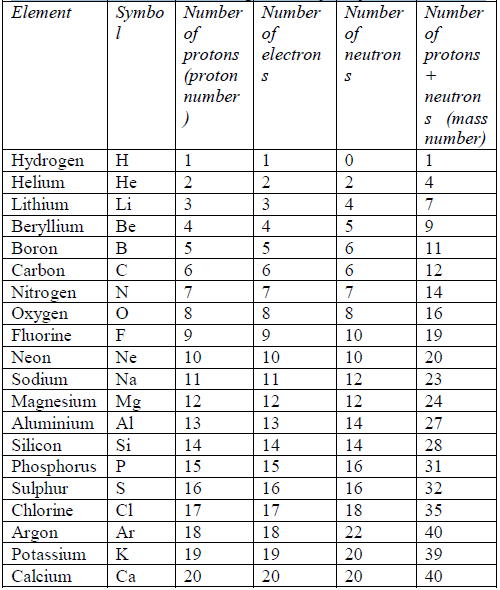The Concept of Isotope
Explain the concept of isotope

Atoms of the same element may have different numbers of neutrons. In a normal situation, atoms of the same element will have the same number of neutrons. However, many cases occur in which two atoms of the same element contain the same number of protons but different numbers of neutrons. Having equal number of protons, these atoms must also have equal numbers of electrons. However, the differing numbers of neutrons cause the atoms to have different mass numbers. An element showing such properties is said to show isotopy and the varieties of the atom are called isotopes of the element.

Therefore, isotopy can be defined as the tendency of atoms of one element to posses the same atomic number but different mass numbers (atomic masses). Isotopes can be defined as atoms of the same element with the same number of protons but different numbers of neutrons, or as „atoms of the same element with the same atomic number but different atomic masses‟.

The isotopes of an element have the same chemical properties because they contain the same number of electrons. It is the number of electrons in an atom that decides the way in which it forms bonds and reacts with other atoms. However, some physical properties of the isotopes are different. The masses of the atoms differ, and therefore other properties, such as density and rate of diffusion, also vary.

Many isotopes (like tritium) are unstable. The extra neutrons in their nuclei cause them to be unstable so that nuclei break spontaneously (that is, without any extra energy being supplied), emitting certain types of radiation. They are known as radioisotopes.

Notation for isotopes
In order to distinguish between different isotopes of the same element in writing symbols and formulae, a simple system is adopted. The isotope of an element, say X will have the symbol X, AZ , where A is the mass number of the isotope and Z is the atomic number of any atom of X. Thus, for all isotopes of one element, Z is constant, and A varies because there are different numbers of neutrons in the different isotopes of the element. For example, the three isotopes of carbon are expressed as 12C6, 13C6, and 14C6. Chlorine has two isotopes: 35Cl17 and 37Cl17 . Since A represents the total number of neutrons and protons in the nucleus of an atom (mass number/atomic mass), and because Z is the number of protons (atomic number), then the number of neutrons in the nucleus of a given isotope is given by:Number of neutrons in the nucleus = A – Z

Relative atomic masses
As we have seen, most elements exist naturally as isotopes. Therefore, the value we use for the atomic mass of an element is an average mass. This takes into account the proportions (abundance) of all the naturally occurring isotopes. If a particular isotope is present in high proportion, it will make a large contribution to the average.

Example

A sample of chlorine gas contains 75% of the isotope 35Cl17 and 25% of the other isotope 37Cl17 . What is the relative atomic mass of chlorine?

Solution

To work out this problem, simply multiply the mass number ofeach isotope with the abundance and sum up the products thus: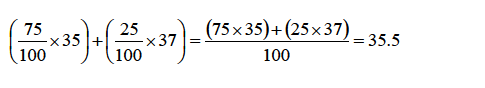This average value for the masses of atoms of an element is known as the relative atomic mass (Ar).Therefore, the relative atomic mass of chlorine is 35.5 (i.e., Ar =35.5).

Select the fields to be shown. Others will be hidden. Drag and drop to rearrange the order.
• Image
• SKU
• Rating
• Price
• Stock
• Availability